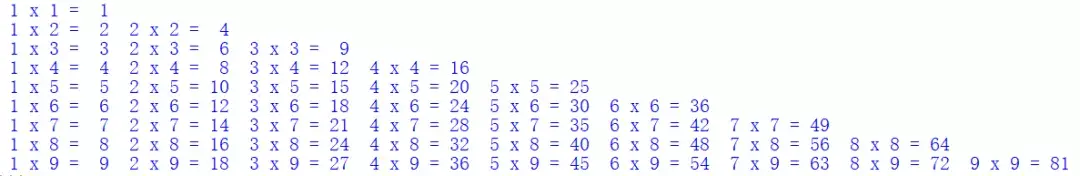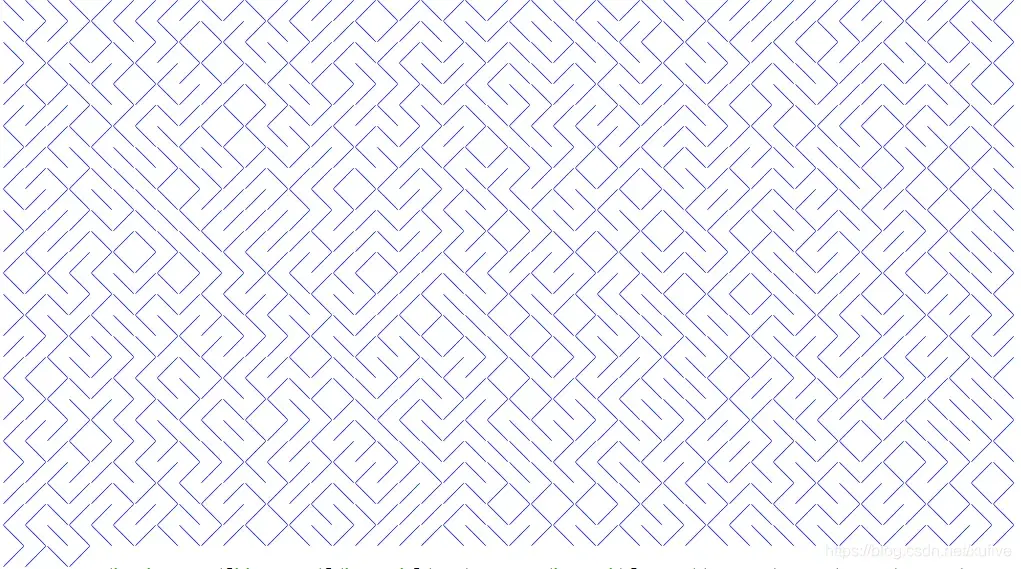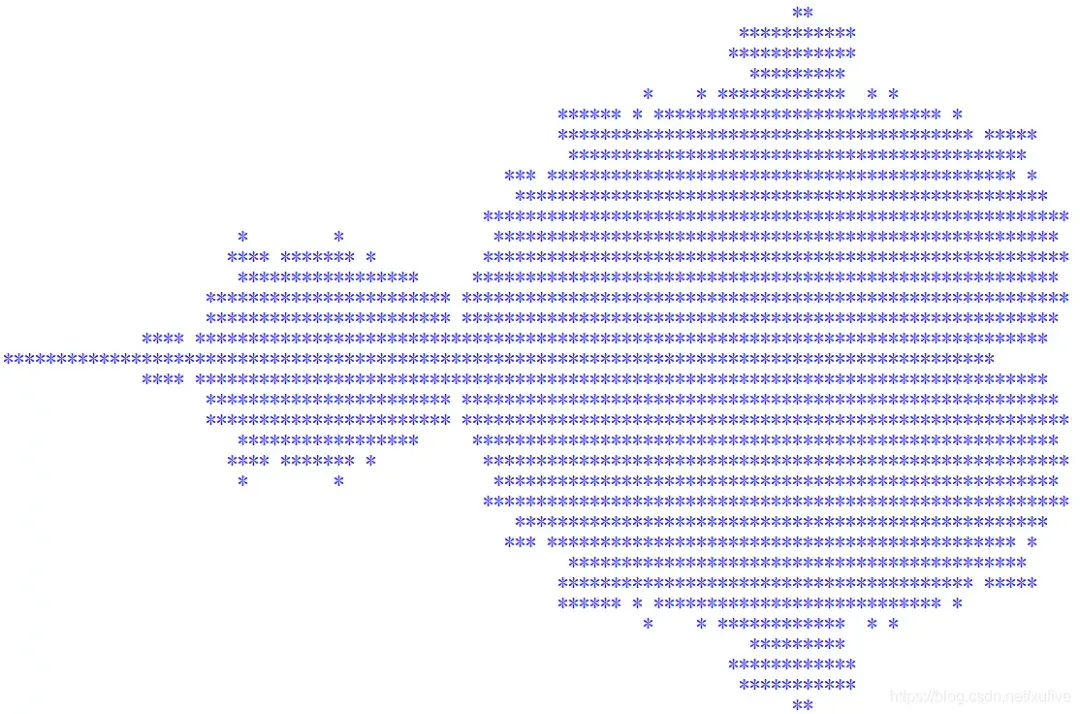一行 Python 代码能实现哪些丧心病狂的功能？（4个案例）这个数据结构，自然是 numpy 的 ndarray 对象，读取图像文件我习惯使用 PIL。因此，需要导入两个模块：

import numpy as npfrom PIL import Image

data = np.stack([np.array(Image.open('head%d.png'%i)) for i in range(109)], axis=0)

data = list()for i in range(109): img = Image.open('head%d.png'%i) img = np.array(img) data.append(img)data = np.stack(data, axis=0)

1. 一行代码打印乘法口诀

print('\n'.join([' '.join(["%2s x%2s = %2s"%(j,i,i*j) for j in range(1,i+1)]) for i in range(1,10)]))2. 一行代码打印迷宫

print(''.join(__import__('random').choice('\u2571\u2572') for i in range(50*24)))3. 一行代码表白爱情

print('\n'.join([''.join([('Love'[(x-y) % len('Love')] if ((x*0.05)**2+(y*0.1)**2-1)**3-(x*0.05)**2*(y*0.1)**3 <= 0else' ') for x in range(-30, 30)]) for y in range(30, -30, -1)]))4. 一行代码打印小龟龟

print('\n'.join([''.join(['*' if abs((lambda a:lambda z,c,n:a(a,z,c,n))(lambda s,z,c,n:z if n==0 else s(s,z*z+c,c,n-1))(0,0.02*x+0.05j*y,40))<2 else ' ' for x in range(-80,20)]) for y in range(-20,20)]))你在 python 使用过程中用一行代码实现过哪些激动人心的功能呢？